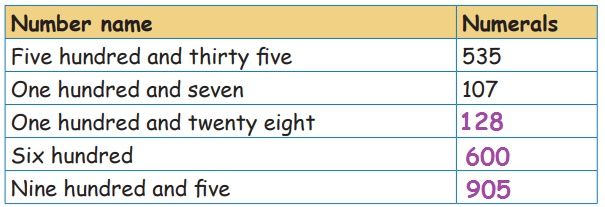Home | | Maths 3rd Std | Read and write all three digit numbers and number names

# Read and write all three digit numbers and number names

Teacher’s note : Let the children explore to represent many 3 digit numbers by using math kit.

Read and write all three digit numbers and number names.

We shall represent 100 in an abacus as shown below.No beads in the ones place shows 0 ones.

No beads in the tens place shows 0 Tens.

1 bead in the hundred place shows 1 hundred.

The place value higher than tens place is hundreds place.

Hundred (or) 100 is the smallest three digit number.

Examples:Activity 1

10 ones = 1 Ten

10 Tens =1 Hundred

10 Hundreds = 1 Thousand.Teacher’s note : Let the children explore to represent many 3 digit numbers by using math kit.

Read and write the numbers from 101 to 200.Teacher‛s Note : Teacher can give practice to children to write the numbers upto 1000.

The number name of the numeral 101 is written by adding one hundred with one as one hundred and one. For the numeral 199 it is written as one hundred and ninety nine.

Activity 2

Write the numerals for the given number names.Activity 3

Write the number names for the following Numerals.Activity 4

Form three digit numbers using each of the given numbers only once.Place value of a numeral in the given number.

write the place value of the underlined digit in the given number.I. find the numbers represented in the abacus by writing their place value.3 – Hundreds

4 – Tens

2 – Ones

300 + 40 + 2

Ans: 3424 – Hundreds

2 – Tens

1 – Ones

400 + 20 + 1

Ans: 4211 – Hundreds

5 – Tens

3 – Ones

100 + 50 + 3

Ans: 153

II. Expand the given numbers into ones tens and hundredsIII. Write the simplified form of the number of the given expansions.Skip counting starting from any given number.

Example:1. 10 11 12 13 14 15 → Skip counted in ones

2. 62 64 66 68 70 72 → Skip counted in twos

complete the following by skip counting in 5s ,10s and 100s.

1. 250 255 260 265 270

2. 500 510 520 530 540

3. 100 200 300 400 500 600Tags : Numbers | Term 1 Chapter 2 | 3rd Maths , 3rd Maths : Term 1 Unit 2 : Numbers
Study Material, Lecturing Notes, Assignment, Reference, Wiki description explanation, brief detail
3rd Maths : Term 1 Unit 2 : Numbers : Read and write all three digit numbers and number names | Numbers | Term 1 Chapter 2 | 3rd Maths